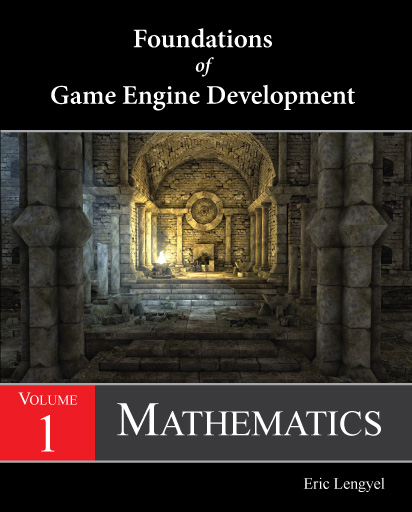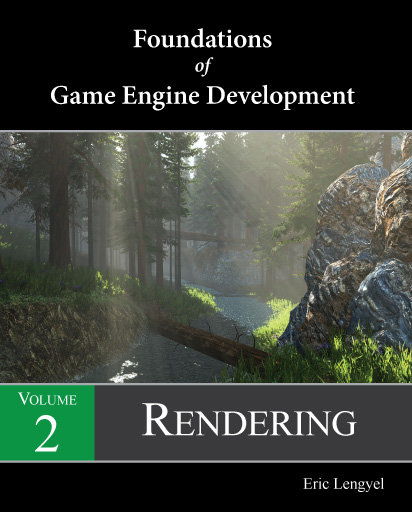﻿ Foundations of Game Engine Development Errata

# Game Engine Development

This is the errata page for the Foundations of Game Engine Development book series. Corrections are listed by volume and printing. To find out which printing you have, look on the copyright page. The code on this website has been updated to include all of the corrections below.Volume 1: Mathematics Eighth Printing and Earlier Page 157. In both Equations (4.42) and (4.43), the coefficient for the last term should be (axby − aybx). Seventh Printing and Earlier Page 120. In Listing 3.6, the return type for the function should be `bool`. Sixth Printing and Earlier Page 46. The penultimate line of the second paragraph should begin with “test that can be used to determine”. Fifth Printing and Earlier Page 162. Every term in the right side of Equation (4.58) should be negated. It's still the same line, but it points in the opposite direction. Page 169. Related to previous item. In Listing 4.1, each component of the returned line should be negated. Fourth Printing and Earlier Page 92. In the second line preceding Equation (2.72), the reference to Equation (2.70) should instead be a reference to Equation (2.71). Third Printing and Earlier Page 21. The end of the sentence preceding Equation (1.33) should read “then the dot product can also be expressed as”. Second Printing and Earlier Page 18. The third line following Equation (1.28) should begin with “v = (0,0,1)”. First Printing Page 21. In the caption for Listing 1.6, the listing number should be set in bold type. Page 45. In the paragraph that begins with “Steps A and B are called pivoting”, the fourth line should read “by choosing the largest entry in each column because this causes the scalar factors”. Page 65. In Equation (2.23), all of the off-diagonal elements in the left matrix should be negated. For example, the (0,1) and (1,0) entries should both be −axay.Volume 2: Rendering Ninth Printing and Earlier Page 263. In Equation (9.16), the three occurrences of the vector v on the right side of the equation should be normalized to unit length and written as v̂. Page 264. Related to previous item. In Listing 9.17, the first three terms in the calculation of `m` should be divided by the magnitude of `v` as in the following code. ```bool OrientedBoxIlluminated(const Point3D& lightPosition, float rmax, const Point3D& center, const Vector3D& size, const Vector3D *axis) { Vector3D v = center - lightPosition; float vs = fabs(Dot(v, axis)); float vt = fabs(Dot(v, axis)); float vu = fabs(Dot(v, axis)); float v2 = Dot(v, v); float m = (size.x * vs + size.y * vt + size.z * vu) * rsqrt(v2) + rmax; if (v2 >= m * m) return (false); return (fmax(fmax(vs - size.x, vt - size.y), vu - size.z) < rmax); }``` Eighth Printing and Earlier Page 172. In the third line after Equation (8.55), “is output” should be “are output”. Page 178. In Listing 8.8, the first texture fetch should have `lightCoord` and `depth` as separate parameters to be consistent with the rest of the section. Seventh Printing and Earlier Page 119. In Listing 7.5, The expression assigned to `nz` should be `1.0F / sqrt(dx * dx + dy * dy + 1.0F)`. Sixth Printing and Earlier Page 364. In Listing 10.17, each `colorTexture` should be `colorBuffer`. Page 367. In Listing 10.18, each `colorTexture`, `structureTexture`, and `velocityTexture` should be `colorBuffer`, `structureBuffer`, and `velocityBuffer`. Page 373. In the last sentence of the second paragraph, replace “is determined” with “are each determined”. Page 378. In the middle of the third paragraph, replace “is the x, y, and z directions” with “in the x, y, and z directions”. Page 380. In Listing 10.20, parameters of type `int` should have type `int32`. Page 386. In Listing 10.22, for the `ReuseEdgeVertex()` function, the parameter `const CellStorage *const (& deckStorage)` should be `CellStorage *const (& deckStorage)`. Page 388. In Listing 10.23, parameters of type `int` should have type `int32`. Page 388. In Listing 10.23, the third line from the bottom of the page should read `uint16 vcode = vertexCode[a];`. Page 390. In Listing 10.23, near the end of the listing, each `meshTriangle[a].index` should be replaced with `meshTriangle[a].vertexIndex`. Page 391. In Listing 10.24, in the three `for` statements, the loop conditions `i < n`, `j < m`, and `k < h` should be `i < n - 1`, `j < m - 1`, and `k < h - 1`. Fifth Printing and Earlier Page 78. The second sentence on the page should begin with “This is due to the fact”. Page 119. In Listing 7.5, the line on which `nz` is defined should read as follows: `float nz = 1.0F / sqrt(dx * dx + dy * dy + 1.0F);` Page 211. In the caption for Figure 8.21, it should say that the point light is near the upper-left corner of the image, not the upper-right corner. Page 277. The last sentence of the first paragraph should end with “on the other side of the portal to receive any light”. Page 282. The second sentence of the third paragraph should begin with “However, we do not need to create”. Page 307. The last line on the page should begin with “placed using calculations similar to”. Page 310. The line preceding Equation (10.20) should end with “all we have to do is calculate”. Page 313. The eighth line in the paragraph following Figure 10.9 should begin with “Since the change in z coordinate tends to be very small”. Page 320. In the paragraph containing Equation (10.31), the word “the” should be removed near the beginning of the fourth line. Page 341. The last sentence on the page should begin with “For a view frustum having projection distance”. Page 343. In the paragraph following Equation (10.65), the word “the” should be removed at the beginning of the fourth line. Page 353. In Listing 10.13, to be consistent with the HLSL convention used in Listing 8.9, the two lines that sample from the shadow map cascades should both read as follows: `atm += texture(shadowTexture, sampleCoord.xyz, sampleCoord.w) * weight;` Page 355. The third sentence in Section 10.7 should begin with “This causes a phenomenon”. Page 357. In the 11th line from the end of the page, the sentence beginning with “The demonstrates how” should read “This demonstrates how”. Page 365. In the caption to Figure 10.64, the sentence describing part (c) should begin with “Rejecting samples based on large differences in depth can cause surfaces”. Fourth Printing and Earlier Page 114. In Listing 7.4, the identifier `position` should be `vertexArray`, the identifier `texcoord` should be `texcoordArray`, and the identifier `triangle` should be `triangleArray`. Page 119. In Listing 7.5, the identifiers `size.x` and `size.y` should be `width` and `height`. Page 126. The beginning of the second line after Equation (7.52) should read“product. First, it includes the height scale”. Page 132. In Listing 7.10, on the 7th line from the end, the code should be dividing by the angle count instead of multiplying. The whole line should read as follows: `ambientMap[x] = pow(sum * (1.0F / float(kAngleCount)), ambientPower);` Page 144. In the second line following Equation (8.6), it should read “values from being generated”. Page 170. In the first line of Section 8.3.1, “present” should be “presents”. Page 178. The fifth line from the bottom of the page should begin with “this turns out”. Page 178. The last sentence on the page should begin with “Even if such”. Page 183. In the first line after Equation (8.70), remove the word “from”. Page 249. In Listing 9.10, the last return statement has an extra opening parenthesis. It should read as follows: `return (Vector3D(0.0F, v.z, −v.y));` Page 250. In Listing 9.11, the `u` on the third line of page 250 should be a `t`. The whole line should read as follows: `float d = Dot(t, vertex[i]);` Page 261. In the fifth line of the last paragraph, remove the word “the”. Page 266. The third line before Equation (9.19) should begin with “that they are”. Page 267. In Listing 9.18, near the top of the listing, `polyhedron->planeCount` should be `polyhedron->faceCount`, and `cameraPolyhedron` should be `polyhedron`. Third Printing and Earlier Page 8. The end of the fourth line should read “but it also occurs”. Second Printing and Earlier Page 77. In Listing 6.5, the last row of the matrix should be `0.0F, 0.0F, 0.0F, 1.0F`. First Printing Page 182. In Equation (8.66), both minus signs should be plus signs. Page 184. Related to previous item. In Equation (8.73), both minus signs should be plus signs. Page 286. There is a bug in the last part of Listing 9.20. The final loop should be the following: ```for (int32 i = 0; i < vertexCount; i++) { bool cull = false; int32 j = i & 1; const Vector3D *v2 = &vertex[i]; Vector3D planeNormal = Normalize(Cross(*v2, *v1)); for (int32 k = 0; k < 4; k++) { const Vector3D& frustumNormal = frustumPlane[k].GetNormal(); float d = Dot(frustumNormal, *v2); vertexDistance[j ^ 1][k] = d; // Cull edge lying in frustum plane, but only if its extrusion points inward. if ((fmax(d, vertexDistance[j][k]) < kOccluderEpsilon) && (Dot(planeNormal, frustumNormal) > 0.0F)) cull = true; } if (!cull) occluderPlane[planeCount++] = Plane(planeNormal, 0.0F) * McamInverse; v1 = v2; }```